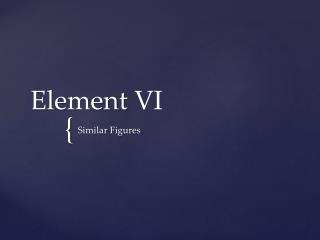DownloadDownload PresentationElement VI

# Element VI

Télécharger la présentation## Element VI

- - - - - - - - - - - - - - - - - - - - - - - - - - - E N D - - - - - - - - - - - - - - - - - - - - - - - - - - -
##### Presentation Transcript

1. Element VI Similar Figures

2. Definition 1 Similar rectilinear figures are such as have their angles severally equal and the sides about the equal angles proportional. • Definition 2Two figures are reciprocally related when the sides about corresponding angles are reciprocally proportional. • Definition 3A straight line is said to have been cut in extreme and mean ratio when, as the whole line is to the greater segment, so is the greater to the less. • Definition 4The height of any figure is the perpendicular drawn from the vertex to the base. Definitions

3. Proposition 9 To cut off a prescribed part from a given straight line. • Proposition 10To cut a given uncut straight line similarly to a given cut straight line. • Proposition 11To find a third proportional to two given straight lines. • Proposition 12To find a fourth proportional to three given straight lines. • Proposition 13To find a mean proportional to two given straight lines. • Proposition 18To describe a rectilinear figure similar and similarly situated to a given rectilinear figure on a given straight line. • Proposition 25To construct a figure similar to one given rectilinear figure and equal to another. • Proposition 28To apply a parallelogram equal to a given rectilinear figure to a given straight line but falling short by a parallelogram similar to a given one; thus the given rectilinear figure must not be greater than the parallelogram described on the half of the straight line and similar to the given parallelogram. • Proposition 29. To apply a parallelogram equal to a given rectilinear figure to a given straight line but exceeding it by a parallelogram similar to a given one. • Proposition 30To cut a given finite straight line in extreme and mean ratio. Propostitons (VIP)